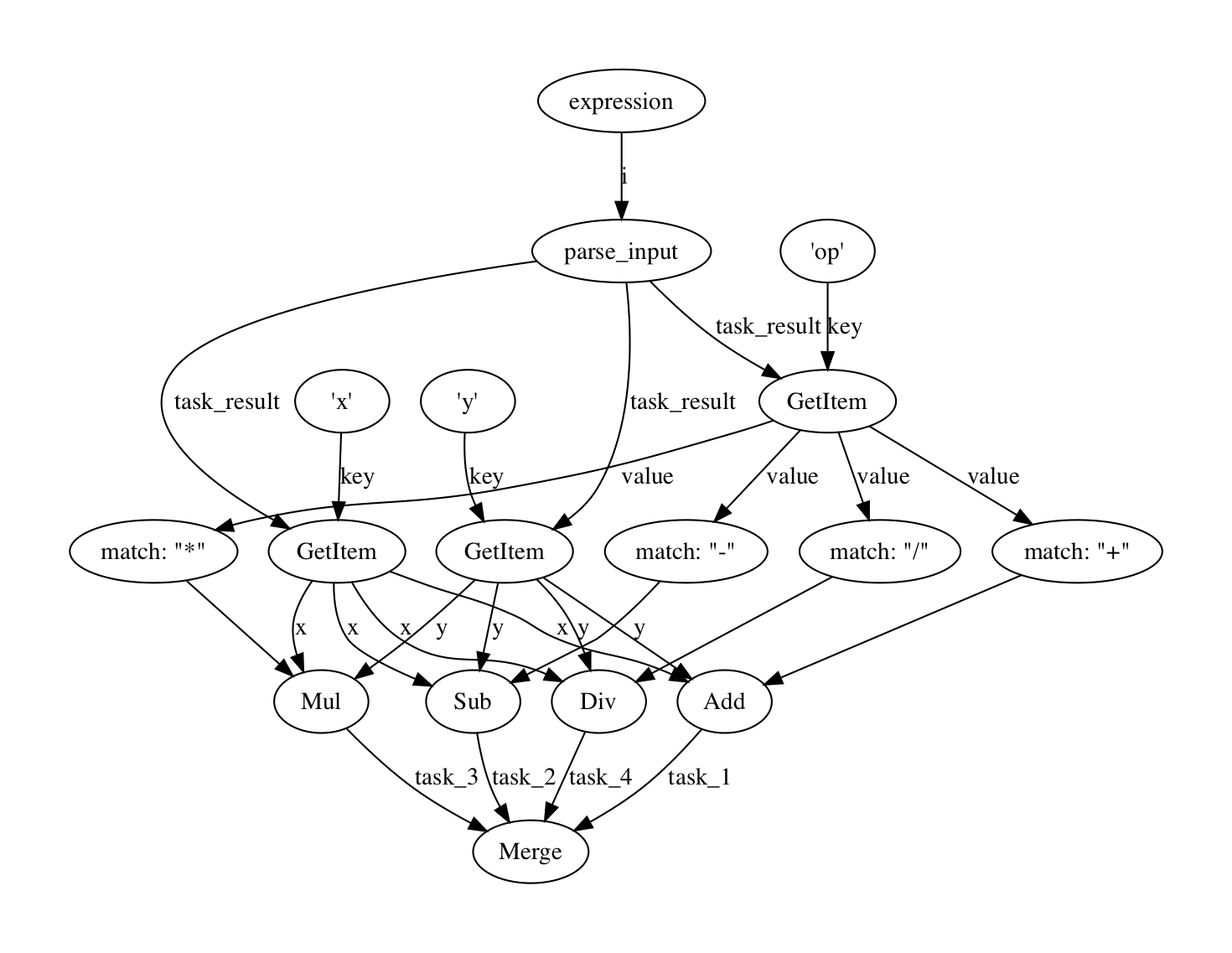# # Using Prefect as a Calculator

Looking for the latest Prefect 2 release? Prefect 2 and Prefect Cloud 2 have been released for General Availability. See https://docs.prefect.io/ for details.

Can your data engineering framework do this?

Prefect is a heavy-duty data workflow system, but it handles lightweight applications just as well.

To illustrate that, let's build a calculator.

## # Setup

Let's write a quick function to make retrieving our calculation results a little easier. All we're going to do is select the value of our terminal task. You don't need to do this, but since we're going to do it a few times in this tutorial, it'll make our examples a little more clear.

``````from prefect import task, Flow, Parameter

def run(flow, **parameters):
state = flow.run(**parameters)
``````

What could be easier than adding 1 to a number?

``````with Flow('Add one') as flow:
result = Parameter('x') + 1
``````

Parameters are like any other task, except that they take their values from user input.

Let's test it out:

``````assert run(flow, x=1) == 2
assert run(flow, x=2) == 3
assert run(flow, x=-100) == -99
``````

Let's kick this up a notch -- why have one input when you could have two?

``````with Flow('Add x and y') as flow:
result = Parameter('x') + Parameter('y')
``````

Multiple Parameters

A flow can have as many parameters as you want, as long as they have unique names.

Our new calculator works like a charm:

``````assert run(flow, x=1, y=1) == 2
assert run(flow, x=40, y=2) == 42
``````

## # Arithmetic

Addition's all very well, but let's give our users some choices. We can combine a new `op` parameter with a `switch` to let users choose the calculation they want done, then combine the results into a single output with `merge`:

``````from prefect.tasks.control_flow import switch, merge

# note: this will raise some warnings, but they're ok for this use case!
with Flow('Arithmetic') as flow:
x, y = Parameter('x'), Parameter('y')
operations = {
'+': x + y,
'-': x - y,
'*': x * y,
'/': x / y
}
switch(condition=Parameter('op'), cases=operations)
result = merge(*operations.values())
``````

Conditional Branches

Prefect has a few ways to run tasks conditionally, including the `switch` function used here and the simpler `ifelse`.

In this case, the `switch` checks for the value of the `op` parameter, and then executes the task corresponding to the appropriate computation. A `merge` function is used to combine all the branches back in to a single result.

Now when we run our flow, we provide the desired operation:

``````assert run(flow, x=1, op='+', y=2) == 3
assert run(flow, x=1, op='-', y=2) == -1
assert run(flow, x=1, op='*', y=2) == 2
assert run(flow, x=1, op='/', y=2) == 0.5
``````

## # Parsing input

Our arithmetic calculator works, but it's a bit cumbersome. Let's write a quick custom task to take a string expression and parse it into our `x`, `y`, and `op`; the rest of the code is the same as before:

``````@task
def parse_input(expression):
x, op, y = expression.split(' ')
return dict(x=float(x), op=op, y=float(y))

with Flow('Arithmetic') as flow:
inputs = parse_input(Parameter('expression'))

# once we have our inputs, everything else is the same:
x, y = inputs['x'], inputs['y']
operations = {
'+': x + y,
'-': x - y,
'*': x * y,
'/': x / y
}
switch(condition=inputs['op'], cases=operations)
result = merge(*operations.values())
``````

The `@task` decorator is a simple way to turn any function into a task.

Just as we've shown that tasks can be added (or subtracted, or multiplied, or divided), they can be indexed as well. Here, we index the result of the `inputs` task to get `x`, `y`, and `op`. Like every other Prefect operation, the indexing itself is recorded in the computational graph, but the execution is deferred until the flow is run and the indexed result is actually available.

And now we can run our calculator on string expressions 🎉:

``````assert run(flow, expression='1 + 2') == 3
assert run(flow, expression='1 - 2') == -1
assert run(flow, expression='1 * 2') == 2
assert run(flow, expression='1 / 2') == 0.5
``````

For the curious and/or brave, here's a visualization of the computational graph Prefect automatically tracked and generated:

``````flow.visualize()
``````